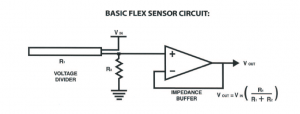# Arduino Sensor Applications with Flex

0
2149### Introduction

we have made in this article with a simple Arduino you flex (flexibility) sensor will let the oil according to the state of the analog value of the applications received from flex sensor.

‘Flex Sensor’ or ‘Bend Sensor’ is a sensor that changes its resistance depending on the amount of bend on the sensor.

They convert the change in bend into electrical resistance – the more the bend, the more the resistance value.

Flex sensors are widely used in various applications such as – Automotive controls, Medical Devices, Fitness Products, Virtual Reality gaming consoles, Animatronics, Robotics, etc

A flex sensor to measure, well, flex! A flex sensor uses carbon on a strip of plastic to act like a variable resistor, but instead of changing the resistance by turning a knob, you change it by flexing (bending) the component. We use a “voltage divider” again to detect this change in resistance.

The sensor bends in one direction and the more it bends, the higher the resistance gets; it has a range from about 10K ohm to 35K ohm. In this circuit we will use the amount of bend of the flex sensor to control the position of a servo.

### Used materials

• Arduino UNO R3
• 2.2 “Flex Sensor
• 3 LED diodes
• 10K resistance (flex sensor)
• 220 ohm resistor (for LED diodes)

It seems that the circuit diagram in the image below.### The logic of the flex sensors

Flexibility sensors are bent sensor tanımlanmaktadır.flex in English at the bend or flex (stretch it) when the sensor resistance of the flex sensor resistance on metal pads 2.2 is outside the fold “and 4.5” sizes of flex sensors as often we can make this sensor in ourselves.

Below is a simple flex sensor circuit is provided.

###Arduino Code:

`#define green 2`
`#define red 3`
`#define yellow 4`
`void setup()`
`{`
`pinMode(yellow,OUTPUT);`
`pinMode(green,OUTPUT);`
`pinMode(red,OUTPUT);`
`Serial.begin(9600);`
`}`
`void loop()`
`{`
`int sensor, degrees;`
`sensor = analogRead(0);`
`degrees = map(sensor, 768, 853, 0, 90);`
`Serial.``print``(``"analog input: "``);`
`Serial.``print``(sensor,DEC);`
`Serial.``print``(``" degrees: "``);`
`Serial.println(degrees,DEC);`
`if``(sensor<580)`
`{`
`digitalWrite(yellow,LOW);`
`digitalWrite(green,HIGH);`
`digitalWrite(red,LOW);`
`}`
`else` `if``((sensor>580)&&(sensor<650))`
`{`
`digitalWrite(green,LOW);`
`digitalWrite(yellow,HIGH);`
`digitalWrite(red,LOW);`
`}`
`else`
`{`
`digitalWrite(red,HIGH);`
`digitalWrite(green,LOW);`
`digitalWrite(yellow,LOW);`
`}`
`delay(100);`
`}`
SHARE
Previous articlePlain carbon steel
Next articleAlloy steel